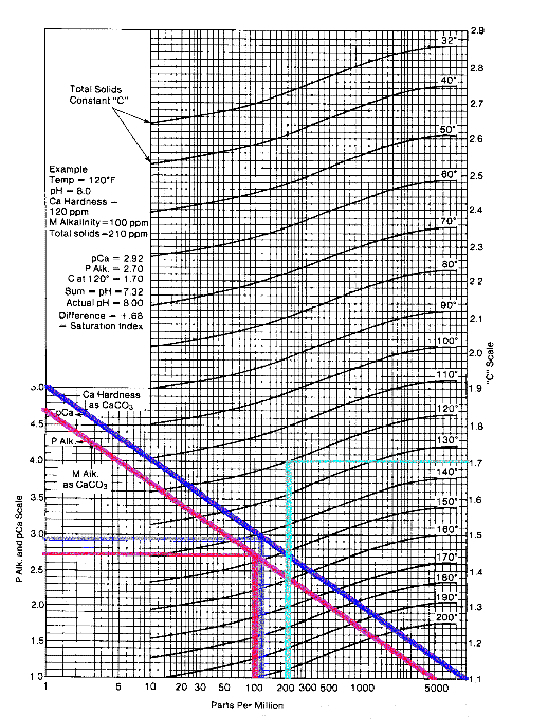•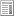• home
•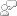• contact us
•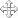• sitemap
Monday, January 10, 2011 1:38 PM
Knowledge Center# SCALE GUARD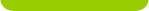# Langelier Saturation Index

Work done by Professor Langelier, published in 1936, deals with the conditions at which a given water is in equilibrium with calcium carbonate. The equation developed by Langelier makes it possible to predict the tendency of water either to precipitate or to dissolve calcium carbonate. The equation expresses the effects of pH, calcium, total alkalinity, dissolved solids and temperature as they relate to the solubility of calcium carbonate for waters in the 6.5 - 9.5 pH range. The equation is written:

### pHs = (pK2 - pKs) + pCa + pAlk.

The left side of the equation represents the pH at which water with a given calcium content and alkalinity is in equilibrium with calcium carbonate. The terms K2 and Ks symbolize the second dissociation constant and the solubility product constant for calcium carbonate, respectively. These terms are functions of temperature and total mineral content. Their values for any given condition can be computed from the known thermodynamic constants. Both the calcium and the alkalinity terms are the negative logarithms of their respective concentrations. The calcium content is molar, while the alkalinity is an equivalent concentration. That is, it is the titratable equivalence of alkaline base per liter.

The algebraic difference between the actual pH of a sample of water and its computed pHs is called the Calcium Carbonate Saturation Index. Hence, Saturation Index equals pH minus pHs.

### Langelier Index (LSI)= pH - pHs

This index is a qualitative indication of the tendency of calcium carbonate to deposit or dissolve. If the index is positive, calcium carbonate tends to deposit (Scale forming). If it is negative, calcium carbonate tends to dissolve (Corrosive). If it is zero, the water is at equilibrium.

The LSI was not intended as an indicator of corrosivity towards mild steel or other metals of construction. The LSI describes only the corrosivity of a water towards an existing calcium carbonate scale, or other calcium carbonate bearing structure.

The calculation of pHs has been simplified by the preparation of various monographs. A suitable one is shown in the picture of the image below.

### pHs is the pH at saturation in calcite or calcium carbonate and is defined as: pHs = (9.3 + A + B) - (C + D)

Where:

A = (Log10 [TDS] - 1) / 10

B = -13.12 x Log10 (oC + 273) + 34.55

C = Log10 [Ca2+ as CaCO3] - 0.4

D = Log10 [alkalinity as CaCO3]